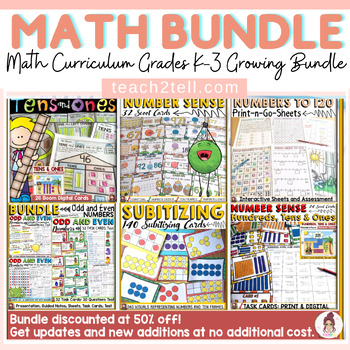DID YOU KNOW:
Seamlessly assign resources as digital activities

Learn how in 5 minutes with a tutorial resource. Try it Now

Learn More# MATH CURRICULUM: GRADES K-3: GROWING BUNDLEK - 3rd, Homeschool
Subjects
Standards
Resource Type
Formats Included
• Zip
• Internet Activities
Pages
872 SHEETS; 360 TASK CARDS; 126 SLIDES; 520 BOOM DIGITAL CARDS + DIGITAL GOOGLE SLIDES & GOOGLE FORM
\$41.00
Bundle
List Price:
\$81.75
You Save:
\$40.75
\$41.00
Bundle
List Price:
\$81.75
You Save:
\$40.75This bundle contains one or more resources with Google apps (e.g. docs, slides, etc.).

#### Products in this Bundle (17)

showing 1-5 of 17 products

#### Bonus

BONUS RESOURCES X 13

### Description

⭐RESOURCES ADDED TO THE BONUS FILE

Fraction: Fifths

Fractions: Sixths

Equivalent Fractions: Using Fraction Bars (added 2021)

Math Digital (Boom Cards) Test Prep 1 (added 2021)

Naplan Prep Tests 1-3 (Aus) (Print & Google Slides - added 2021)

Naplan Prep Tests 1-3 (Aus) (Boom Decks - added 2021)

Math Digital (Boom Cards) Test Prep 3 (added 2021)

--------------------------------------------------------------------------------------------------

✅ Get access to all future additions and updates for this growing bundle. This bundle is now discounted at 50% off! (the maximum discount allowed on the site)

Get each pack for less than \$2.50! +BONUS!

✅ Given that students in a cohort differ in academic aptitude, the resources in this bundle best target learning skills for students in grades K-3.

✅ All additional resources will be added to this bundle as and when they are created.

--------------------------------------------------------------------------------------------------------------------------

Find 4-7 Math Curriculum Bundle below:

--------------------------------------------------------------------------------------------------------------------------

Total Pages
872 SHEETS; 360 TASK CARDS; 126 SLIDES; 520 BOOM DIGITAL CARDS + DIGITAL GOOGLE SLIDES & GOOGLE FORM
Included
Teaching Duration
N/A
Report this Resource to TpT
Reported resources will be reviewed by our team. Report this resource to let us know if this resource violates TpT’s content guidelines.

### Standards

to see state-specific standards (only available in the US).
Compare two fractions with the same numerator or the same denominator by reasoning about their size. Recognize that comparisons are valid only when the two fractions refer to the same whole. Record the results of comparisons with the symbols >, =, or <, and justify the conclusions, e.g., by using a visual fraction model.
Recognize and generate simple equivalent fractions, (e.g., 1/2 = 2/4, 4/6 = 2/3). Explain why the fractions are equivalent, e.g., by using a visual fraction model.
Understand two fractions as equivalent (equal) if they are the same size, or the same point on a number line.
Explain equivalence of fractions in special cases, and compare fractions by reasoning about their size.
Apply properties of operations as strategies to multiply and divide. Examples: If 6 × 4 = 24 is known, then 4 × 6 = 24 is also known. (Commutative property of multiplication.) 3 × 5 × 2 can be found by 3 × 5 = 15, then 15 × 2 = 30, or by 5 × 2 = 10, then 3 × 10 = 30. (Associative property of multiplication.) Knowing that 8 × 5 = 40 and 8 × 2 = 16, one can find 8 × 7 as 8 × (5 + 2) = (8 × 5) + (8 × 2) = 40 + 16 = 56. (Distributive property.)